﻿ Testing the Consistency of Subjective Weights in Goal Programming – the Analytical Hierarchy Process Approach

### Testing the Consistency of Subjective Weights in Goal Programming – the Analytical Hierarchy Process...

T. Ganesh, PRS ReddyOPEN ACCESSPEER-REVIEWED

## Testing the Consistency of Subjective Weights in Goal Programming – the Analytical Hierarchy Process Approach

1Department of Food Science and Technology, Pondicherry University, Puducherry, India

2Department of Statistics, Sri Venkateswara University, Tirupati, India

### Abstract

In the context of multi-criteria decision making every individual will act as a decision maker. The decision maker is involved in assigning preferences or weights to the alternatives. Goal programming will also come under the hub of MCDA, where the DM will assign the subjective weights to each goal. By giving so, the weighted sum of deviations will be minimized to some extent but one cannot ascertain whether the weights are consistent or not. In this paper the authors have proposed a simpler way of testing the consistency of weights and demonstrated an easier way to achieve the minimized weighted sum of deviations through AHP. This attempt showed better results and allows the DM to think well before giving the weights to each goal.

• Ganesh, T., and PRS Reddy. "Testing the Consistency of Subjective Weights in Goal Programming – the Analytical Hierarchy Process Approach." American Journal of Applied Mathematics and Statistics 2.3 (2014): 92-95.
• Ganesh, T. , & Reddy, P. (2014). Testing the Consistency of Subjective Weights in Goal Programming – the Analytical Hierarchy Process Approach. American Journal of Applied Mathematics and Statistics, 2(3), 92-95.
• Ganesh, T., and PRS Reddy. "Testing the Consistency of Subjective Weights in Goal Programming – the Analytical Hierarchy Process Approach." American Journal of Applied Mathematics and Statistics 2, no. 3 (2014): 92-95.

 Import into BibTeX Import into EndNote Import into RefMan Import into RefWorks

### 1. Introduction

In a decision making process, the DM plays an important role in defining the preferences for a list of alternatives under each criterion. In such situations, the DM may face complexity in assigning the weights to each alternative. Goal programming is one of the MCDA techniques, where preferences or priorities will matter for each goal. To solve this type of complex (multi-criteria) problems in very simple way, we have one approach by Analytical Hierarchy Process (AHP) proposed by T.L. Saaty (1977, 94). In this paper we highlight the significance of AHP in assigning the weights for each goal, which intern helps in minimizing the weighted sum of deviations.

Considering the structure of goal programming, the goals will act as alternatives and the main objective is to minimize the sum of deviations using the conventional method of solving weighted goal programming, the weights assigned are subjective and depends on the DM. This may lead to subjective conclusions and may not achieve the requirements. To handle this situation we make use of popularly known multi-criteria decision making (MCDM) approach by name AHP. It is a decision support tool which helps in solving complex decision problems. In the recent past, AHP has attracted the interest of many researchers mainly due to the nice mathematical properties.

The AHP is pair wise comparison method and uses multi-level hierarchical structure of objective, criteria and alternatives. It has its wide spread of applications in diversified fields .

The Analytical Hierarchy Process (AHP) was proposed by T.L. Saaty in 1977 [1, 2]. It is very popular approach to solve MCDM problems, which involves qualitative data. The method uses a reciprocal decision matrix obtained by pair wise comparisons. The pair wise comparison method was introduced by Fechner in 1860 and developed by Thurstone in 1927. Based on pair wise comparison, Saaty proposed the analytical hierarchy process (AHP) as a method for multi-criteria decision making . It provides a way of breaking down the general method into a hierarchy of sub-problems, which are easier to evaluate. For these pair wise comparisons, criteria and sub criteria of their alternatives are measured using the eigenvector method . The geometric mean method has been used instead of eigenvector method for estimating the weights for judgment matrix . For testing the consistency of weights in comparison matrix, the following three methods are existing in the literature such as positive reciprocal matrix [3, 5, 7], the Logarithmic Least Square Method (LLSM) and least squares method (LSM)  can be used. For each and every method the ranks will be assigned to the pair wise comparisons of the judgment matrix , further a scaling method has been derived to ascertain the priorities in AHP . Bradely – Terry model  illustrates the importance of the eigenvector method and geometric mean method for deriving the priorities. Two new improvised approaches were proposed, which helps in a proper and optimum allocation of weights in a weighted goal programming problem .

### 2. Methodology

The DM’s are experts will give preferences in the form of pair wise comparison matrices and these can be represented in pairs. In this comparison matrix, each element is an upper level is used to compare the elements in the level immediately below with respect to it. The priorities obtained from the comparisons to weigh the priorities in the level immediately below. This process is to be continued for every element. Then for each element in the level below add its weighted values and obtained its overall priority. This process of weighing and adding is to be continued until the final priorities of the alternatives in the bottom most level are obtained. Doing comparisons, we need a scale of numbers that indicates how many times more important element is over other element with respect to the criterion with respect to which they are compared. The fundamental scales of absolute numbers are given below.

#### Table 1. Scale of absolute measures

Let A1, A2, …, An be the ‘n’ comparable alternatives with w1, w2, …, wn as its weights. The matrix of ratios of all weights is given below.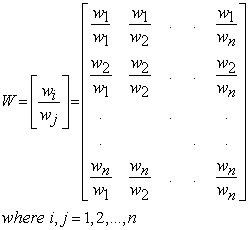The matrix of pair wise comparisons A = [aij] represents in the intensities of the DM’s preference between individual pairs of alternatives (Ai versus Aj, for all i, j = 1, 2,…, n). They are usually chosen from a given scale (1/9, 1/8, 1/7, 1/6, 1/5, ¼, 1/3, ½, 1, 2, 3, 4, 5, 6, 7, 8, 9). Given n alternatives {A1, A2, …, An}, a DM compares pairs of alternatives for all the possible pairs and a comparison matrix A is obtained, where the element aij shows the preference weight of Ai obtained by comparison with Aj.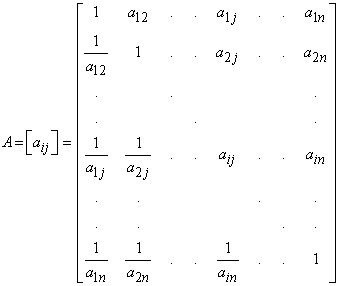If a matrix A is absolutely consistent, we notice that A=w and in the ideal case of total consistency, the principal eigenvalue (λmax) is equal to ‘n’, that is λmax = n, the relations between the weights and judgments will be given byfor i, j = 1,2,…,n. The weights wi, i=1,2,…,n, were obtained using the eigenvector method, they are positive and normalized and satisfy the reciprocal property.

In the matrix ‘A’, aij’s give the relative importance of elements i and j for each entry of the matrix ‘A’ (aij > 0) and reciprocal (1/aij = aij, i, j = 1,2,…,n). In judgment matrix ‘A’, the corresponding maximum left eigenvector is approximated by using the geometric mean (GM) of each row. For GM values, the numbers are normalized by dividing them with their sum. One of the most practical issues in AHP is that it allows for slightly non-consistent pair wise comparisons. A different situation appears when the matrix is neither totally consistent nor contradictory. In this case, Saaty defined the consistency index (CI) which is as follows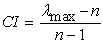If the comparison matrix is completely consistent then aij.ajk = aik (for all i, j, k); λmax = n; CI = 0. In this case, the two different matrices of judgments (A) and weights (w) are equal. If the matrix is not absolutely consistent then λmax > n, and we need to measure the level of inconsistency. Saaty defined the consistency ratio (CR) as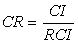The Random Consistency Index (RCI) values for different values of n can be obtained by Saaty (1980). If CR < 0.10, the matrix is consistent, otherwise. If CR > 0.10 re-evaluate the pair wise comparisons and test the consistency by AHP.

Using AHP, we assign the weights in weighted goal programming problem. In the conventional method of weighted goal programming problem, DM has been given the penalty weights with subjective knowledge. This penalty weights will not give any justification and are not consistent. So, we made an attempt to assign the weights by AHP in weighted goal programming problem.

For the penalty weights, DM will decide the comparisons of each pair of the goals. If n goals are available, the total numbers of pair wise comparisons are n (n-1)/2. Using this, we get a comparison matrix of order nxn. Penalty weights are to be tested for consistency. If these weights are consistent, we use these weights in weighted goal programming problem and get better results.

### 3. Numerical Example

Let us consider an example of company, which produces two products popular with home renovators: old-fashioned chandeliers and ceiling fans. Both products require a two-step production process involving wiring and assembly. It takes about 2 hours to wire each chandelier (x1) and 3 hours to wire a ceiling fan (x2). Final assembly of the chandeliers and fans requires 6 and 5 hours, respectively. The production capability is such that only 12 hours of wiring time and 30 hours of assembly time are available. Each chandelier nets the firm \$7.00 and each fan \$6.00 and the company has produce as much profit above \$30.00 as possible during the production period. Fully employ available wiring hours. Avoid overtime in assembly hours. Produce at least seven (7) ceiling fans.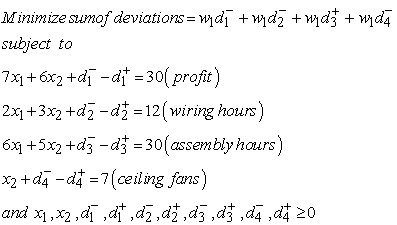Let w = weighted sum of deviations from the goal = number of penalty points incurred by missing the goals

Therefore, the overall objective is to minimize the weighted sum of deviation from the goals. Here, we have assigned some empirical weights to each one of the goal in the objective function which is defined below:

Min w = 5 (amount under goal1) + 7 (amount under goal2) + 3 (amount over goal3) + 6 (amount under goal4)

However, no penalty weights will be included for the goal1, since the target is to exceed and to get more profit and no penalty weights will be included for the goal2 also. Similar kind of frame work is made set for goal3 and goal4. The final solution is obtained by using the conventional weighted goal programming model is reported in Table 2.

#### Table 2. Conventional weighted goal programming problem

In the Table 2, the weighted goal programming problem, x2 (ceiling fans) has to work with 6 hours; profit reached \$36 and should maintain 18 hours for wiring, no need to increase or decrease for assembly hours. For these, the company produced 6 ceiling fans only. The weighted sum of deviations is 6 that is the high weighted sum of deviations.

We use the AHP for assigning the weights for each deviational variable. In given weighted goal programming problem, we have four goals. The AHP for testing the consistency of preferences given by DM, the matrix below displays the weights assigned by DM.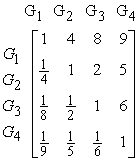The weights of the above derived judgment matrix are 0.63, 0.24, 0.07, 0.05 (sum equal to one) with consistency ratio 0.059, which is less than 0.10. So the given weights are consistent. Then these weights can be used in weighted goal programming problem as weights to deviational variables.Min w = 0.63 (amount under goal1) + 0.24 (amount under goal2) + 0.07 (amount over goal3) + 0.05 (amount under goal4).

#### Table 3. Weighted goal programming problem with penalty weights using AHP

This method (Table 3) gave better results when compared with conventional method (Table 2). The main objective is to minimize the weighted sum of deviations; the same is achieved in terms of level of achievement. The quantities obtained under level of achievement should approach to zero, so that the priorities given by decision maker for the goals by AHP method were well defined. Here the weighted sum of deviations is 0.05, which is equal to zero. Ceiling fans (x2) has to work with 6 hours; profit reached \$36 and should maintain 18 hours for wiring, no need to increase or decrease for assembly hours. For these, the company produced 6 ceiling fans only. Gaining the more profit and selling the more ceiling fans, the DM has to change the weights using the AHP.

### 4. Conclusion

In this paper we have highlighted the advantages of AHP which helps in reducing the complexity and subjective judgment in giving the preferences for a weighted goal programming. Conventionally the weights for the goals are subjective. Using this AHP approach, these subjective weights can be tested for its consistency; the weighted sum of deviations can be further minimized using the Eigen values obtained through AHP approach. Further, the mathematical complexity is simple in AHP approach and allows the beginner to work in a easier way.

### Acknowledge

The first author would like to acknowledge to UGC-BSR for their financial support.

### References

  T.L. Saaty (1977), A scaling method for priorities in hierarchical structures, Journal of mathematical Psychology, 15, pp 234-281.In article CrossRef  T.L. Saaty (1980), the Analytical Hierarchy Process, McGraw – Hill, New York.In article  Jose A.A. and M. Teresa Lamata (2006), Consistency in the AHP process: A new approach, International Journal of uncertainty, fuzziness and knowledge based systems, 14(4), pp 445-459.In article CrossRef  T.L. Saaty (1994), Fundamentals of Decision making and priority theory with the AHP, RWS publications, Pittsburg.In article  J. Barzilai, W.D. Cook and B. Golany (1987), Consistent weights for judgment matrices of the relative importance of alternatives, Operations Research Letters, 6(3), pp 131-134.In article CrossRef  G.B. Crawford (1987), The geometric mean procedure for estimating the scale of judgment matrix, Mathematical Modeling, 9, pp 327-334.In article CrossRef  T.L. Saaty and L.G. Vargas (1984), Inconsistency and rank preservation, Journal of mathematical psychology, 28(2).In article CrossRef  T.L. Saaty (1990), Eigenvector and logarithmic least squares, European Journal of Operations Research, 48, pp 156-160.In article CrossRef  Robert E. Jensen (1984), An alternative scaling method for priorities in hierarchical structures, Journal of mathematical psychology, 28, pp 217-332.In article CrossRef  T.L. Saaty and G. Hu (1998), Ranking by eigenvector versus other methods in the analytic hierarchy process, Applied mathematical letters, 11(4), pp 121-125.In article CrossRef  C. Genest and C.E. M’Lan (1999), Deriving priorities from the Bradley-Terry model, Mathematical and computer modeling, 29, pp 87-102.In article CrossRef  E. Traintaphyllou and Stuart H. Mann (1995), Using the AHP for decision making in the engineering applications: some challenges, International journal of industrial engineering: Applications and practice, 2(1), pp 35-44.In article  T.L. Saaty (2008), Decision making with analytic hierarchy process, International journal of services sciences, 1(1), pp 83-98.In article  T. Ganesh and PRS Reddy (2013), Improved methods for minimizing the weighted sum of deviations using goal programming technique, International Journal of Mathematics and computer applications research, 3(2), pp 115-120.In article